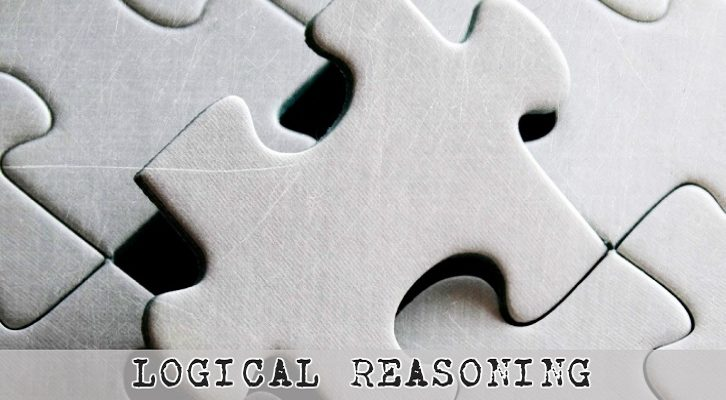# CAT Online Preparation : Interesting one from Logical Reasoning

Consider a cuboid of dimension 6cm * 8cm * 5cm. Three pairs of opposite sides of this cube are painted Red, Green and Blue.  The largest surfaces have been painted Red, the smallest have been painted Blue. This cuboid is subsequently broken down into smaller cubes of dimension 1cm * 1cm * 1cm. Answer the following questions based on the information provided.

1.How many of the smaller cubes have none of their sides painted?

2.How many have exactly 2 sides painted?

3.How many more cubes have red paint as one of their surfaces than the number of ones that have blue paint on them?

This is a question from the online course following which we had given the description “Of course, the solution for this will not be provided. The horse can only be taken to the pond and all that. The extension to this is the question where we deal with a cuboid of side m * n * l with m > n > l.”

Turns out, this question has become akin to the forbidden fruit and the clamour for answers has increased steadily. So, we have decided to have a go at the solution here.

Detailed Explanation

We have been provided with a cube of dimensions 6*8*5, with the faces coloured blue, green and red. The 8*6 surfaces are painted red and the 6*5 surfaces are painted blue. So, the middle 8%5 should have been painted green. In total the cubic units are 240 in number.

Before we go into the solutions, let us define a few terms

1. The cuboid has six surfaces. For the purpose of this question, imagine the room you are in to be the cuboid. The cuboid has 3 pairs of rectangular surfaces around it
2. There are totally 8 * 6 * 5 = 240 smaller cubes that constitute this larger cuboid.
3. Now, we are going to take a count of the different parts of this cuboid.
1. The cuboid has 6 surfaces
2. It has 8 corners. Let us call these as 8 vertices
3. It has 12 straight lines – 4 on the top surface, 4 on the bottom surface and 4 that look like pillars between these two surfaces. Let us call these as “Edges”
4. It has 240 small cubes sitting inside.

Question 1)  How many of the smaller cubes have none of their sides painted?

We are tasked with the determination of the number of colourless cubes; to do this mandates the realization that any cube with at least one face exposed is coloured; thus only cubes hidden from view have to be considered.

The cuboid thus consists of a colourless core and a coloured periphery. The easiest way to do this problem is to understand that the colourless core is itself a cuboid, the dimensions of which are determined by deducting 2 from each of those of the original cuboid enclosing it, this being done to discount the cubes that are exposed, as might be clear from the illustration given below.

Thus the number of uncoloured cubes is 4*6*3 = 72. This is the answer to the first question.

Moving on to the second question –  2)  How many have exactly 2 sides painted?

We have to consider only edge cubes that are not corner cubes—corner cubes have three faces painted—and this is done best by considering one edge at a time from the total of twelve.

There are four edges of length 5; these have but 3 cubes each that are not corner cubes as each edge connects two corners.

There are four edges of length 6; these have but 4 cubes each that are not corner cubes.

There are four edges of length 8; these have but 6 cubes each that are not corner cubes.

Thus the total number in the second category of having two faces painted is 4 times the sum of 3, 4 and 6, which is 52. 4* ( 3 + 4 + 6) = 52.

The third problem is  – 3) How many more cubes have red paint as one of their surfaces than the number of ones that have blue paint on them?

This is easily tackled, as all that is required is the difference between the number of cubes with at least one side red and the number of those with at least one side blue.

For red we have 6*8 + 6*8 = 96.

For blue we have 6*5 + 6*5 = 60.

The difference is thus 36.

The answers are 72, 52 and 36 for the three questions.